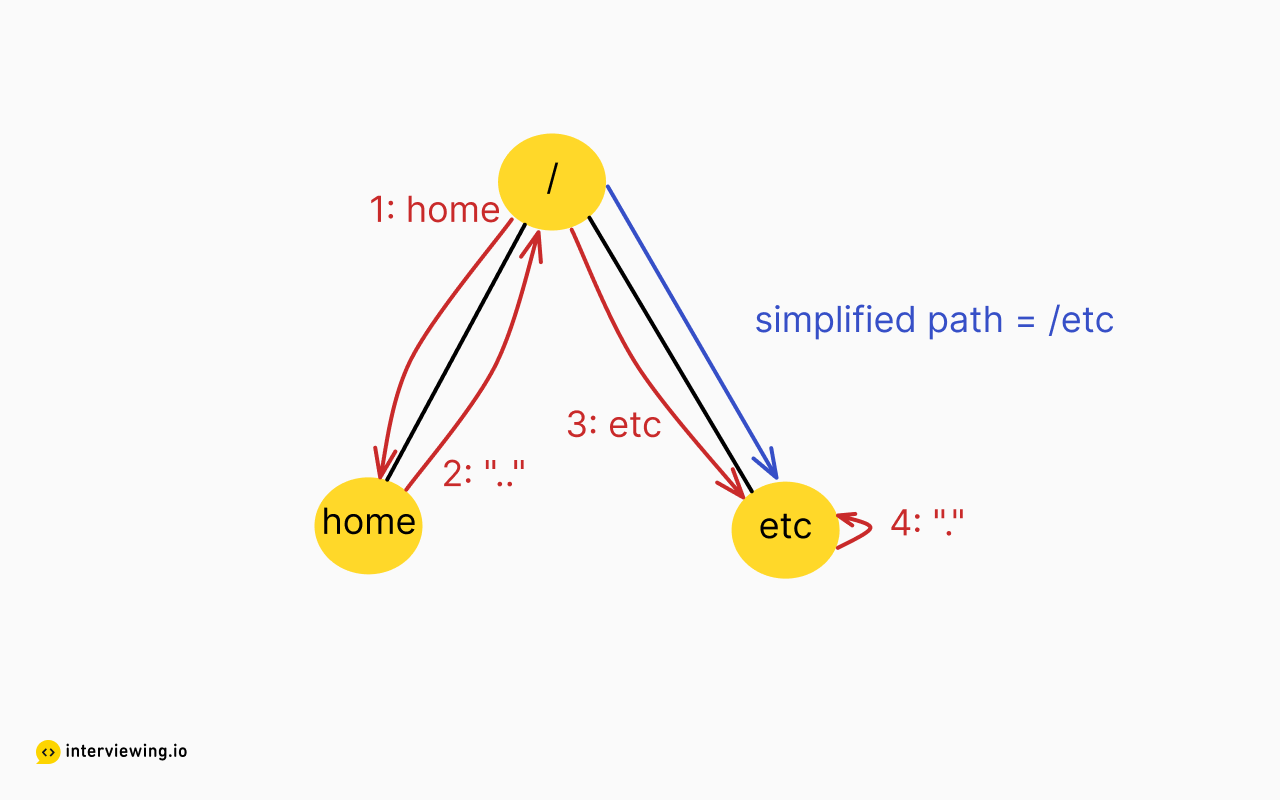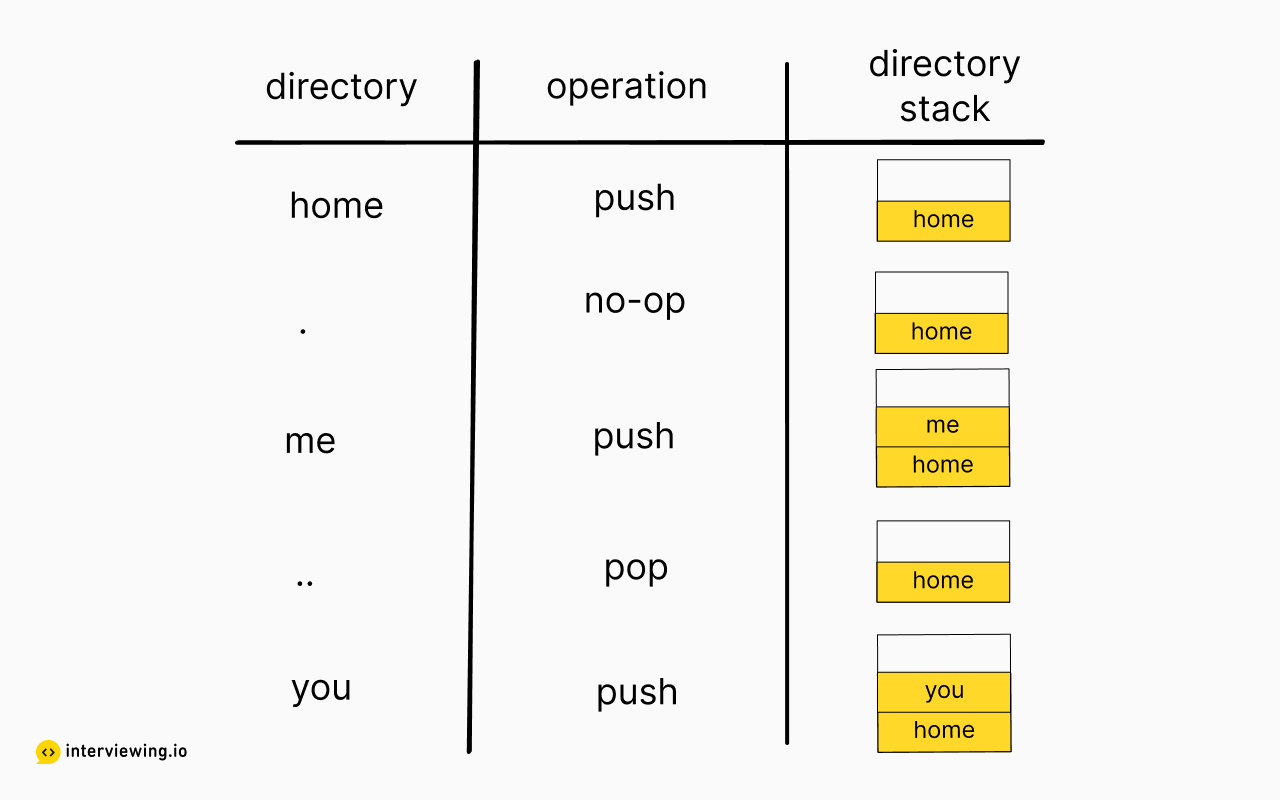MEDIUM
DATA STRUCTURES AND ALGORITHMS

# How to Solve Simplify Path

Written ByTom WagnerDominic Platt

## Simplify Path Introduction

The Simplify Path problem involves taking a path as a string and returning the shortest equivalent path by removing redundant elements such as ".." and ".". This problem can be solved using a variety of techniques such as string manipulation, recursion, or using a stack.

## Simplify Path Problem

You are given a path to a file as a string. The path can contain the symbols: “..” for the parent directory and “.” for the current directory. Convert the path into its simplified form.

### Example Inputs and Outputs

#### Example 1

Input: `path = "/home/../etc"` Output: `"/etc"`

#### Example 2

Input: `path = "/home/./me"` Output: `"/home/me"`

#### Example 3

Input: `path = "/home//me/"` Output: `"/home/me"`

Constraints

• The path is absolute, meaning it always starts with "/"
• `length(path)` > 0
• path is composed of alphanumeric, "." or "/" characters

## Simplify Path Solutions

The path we are given is like any file path on your computer. The file path is made up of a series of directories separated by "/". In addition to regular directories, there are two other symbols: "." which refers to the current directory and ".." which refers to the parent directory. By following each step of the path we can find the file.

Our goal is to simplify the file path. Meaning we need to return the path in its most direct form.

Let's have a look at what simplifying a file path means with a diagram which follows an example of `"/home/../etc/."`We can break down the path into a series of steps separated by "/". Starting at the root directory "/":

1. `"home"`: go to the home directory
2. `".."`: go up one directory - which brings us back to the root directory
3. `"etc"`: go into the etc directory
4. `"."`: remain in the current directory

The simplified path is the direct path to the file. In this case, it is "/etc". Notice how we don't include the "home" directory because we navigated out of it.

With this in mind we can divide our approach into three steps:

1. Parse the path string into a series of directories;
2. Move directory according to each step;
3. Return the direct path to the final directory we are in. Let's break down each step in turn.

#### Step 1: Parse the path string into a series of directories

How can we extract a series of steps from the string? We can see that each directory is separated by a "/" or a series of "/" characters.

Extracting out each directory is then a matter of getting the substring that is in between each "/" character. Most modern programming languages provide utility functions to split a string by a delimiter.

We need to be mindful that consecutive "/" should be considered as a single directory delimiter, and there may or may not be trailing slashes.

#### Step 2: Move directory for each path step

For each directory we encounter we need to move our directory. There are three step types that we need to handle. Each of these we can identify according to the value of the directory step.

1. `"."`: the current directory, don't change directory
2. `".."`: the parent directory, go up one directory
3. Everything else: a genuine directory, go into the directory of that name

How do we record what directory we are currently in? One approach would be to record the current directory as a string. Another option would be to use a stack where each item is a directory. We’ll examine each of these approaches in the solution.

#### Step 3: Return the direct path to the final directory we are in

Depending on the format used for tracking the current directory we may need to convert the path before returning it.

### Approach 1: Directory stack

We utilize the "split" string utility function which returns an array of substrings split by a "/". This allows us to easily convert the path into a series of steps. This has the side effect of generating directories of "" when there are consecutive "/", however we can filter these out and move on to the next directory.

We also use the stack data structure to keep track of our current directory. A stack is a data structure in which items can be added to or removed from the end of the stack in constant time, and in our case the items are directories.

For each directory step, we can push when we need to move into a directory and pop when we need to navigate to the parent directory.

Let's take the example of "/home/./me/../you" and see how we can use a stack to keep track of our current directory.We have been keeping a track of our current directory as a stack. While this is convenient for us as we have been evaluating each step, we need to return the result as a string. To convert our stack to the simplified path we can insert a "/" char in between each item in the stack and return the result.

``````class Solution:
def simplifyPath(self, path):
# Create the stack where we'll keep track of our current directory
pathStack = []

# 1: Convert the path string into directories
for curDir in path.split("/"):
# 2: Move directory for each path step
if not curDir:
# Consecutive "/" will result in empty directories - skip these
# Alternatively, we could filter out duplicate “/” by iterating over string before splitting it up by “/”
pass

elif curDir == ".":
# "." means don't modify current directory
pass

elif curDir == "..":
if pathStack:
# Don't pop stack if we're already at the root directory
pathStack.pop()

else:
# A "genuine" directory, navigate into it
pathStack.append(curDir)

# 3: Return the final directory
return "/" + "/".join(pathStack)
``````

#### Time / Space Complexity

• Time Complexity: `O(n)`, where `n` is the number of characters in the path. Every character of the string is parsed in this solution. The stack push and pop operations take `O(1)` time.
• Space Complexity: `O(n)`, where n is the number of directories in the path. In the worst case, the stack will only be pushed to and so will be of size `O(n)`. This solution also contains the array of directories which will also be of size `O(n)`.

#### Approach 2: Character-by-character parsing

As an alternative to using a stack, we can parse the path string character by character. The current directory is also stored directly as a string rather than using a stack.

As we parse each character there are two cases. The character is a "/" or it isn't. If it isn't a "/" then it is part of a directory so we add it to our current directory string. When we encounter a "/" it means we are at the end of a directory and so we can evaluate the directory. Like before, the loop may produce empty directory strings so we need to make sure to filter those out.

The evaluation of each directory is handled in the same way as the previous method. The main difference is that we store our current directory as a string.

When we need to add a directory we can append the string to our path. When we need to go up a directory the solution is not so straightforward. We need to modify the string so that everything from and including the last "/" is removed. To do that we can locate the index of the last "/" character in the string, we can then slice the string so that everything from that index is removed.

``````class Solution(object):
def simplifyPath(self, path):
# Store the current directory as a string
simplifiedPath = ""
curDir = ""

# Add an ending "/" so that we always evaluate the final directory
path += "/"
for ch in path:
if ch == "/":
if not curDir:
# Consecutive "/" will result in empty directories - skip these
pass
elif curDir == ".":
# "." means don't change directory
pass
elif curDir == "..":
if simplifiedPath:
# Remove the last dir by locating the last "/" char
i = simplifiedPath.rfind("/")
simplifiedPath = simplifiedPath[:i]

else:
# A "genuine" directory, navigate into it
simplifiedPath += "/" + curDir

# Reset the directory string
curDir = ""

else:
curDir += ch

if not simplifiedPath:
# Return the root directory if the final path is empty
return "/"

return simplifiedPath
``````

#### Time / Space Complexity

• Time Complexity: `O(n)`, where `n` is the number of characters in the path. As in the previous solution, every character of the string is parsed. Within the loop, we are calculating the position of the last “/” character. At first glance, it appears this could make the solution `O(n^2)`. However, each time we do this calculation we are only evaluating the last directory string and then removing that directory from the path. In the worst case we’d only be iterating over the entire path again. This makes the solution not as fast as the previous but in terms of time complexity it is still linear.
• Space Complexity: `O(1)`. Disregarding the output and input strings all the other variables have constant space. This assumes the length of a directory is much less than the size of the path itself.

#### Approach Comparison

While both approaches have linear time complexity, the latter solution is not as fast. This is because going up one directory requires us to reparse the string which is not as fast as popping from the stack. However, the latter solution has constant space complexity because it does not require any extra memory.

Verbally noting these tradeoffs in an interview can be a great way to signal your knowledge to the interviewer.

## Practice the Simplify Path Problem With Our AI Interviewer

Start AI Interview